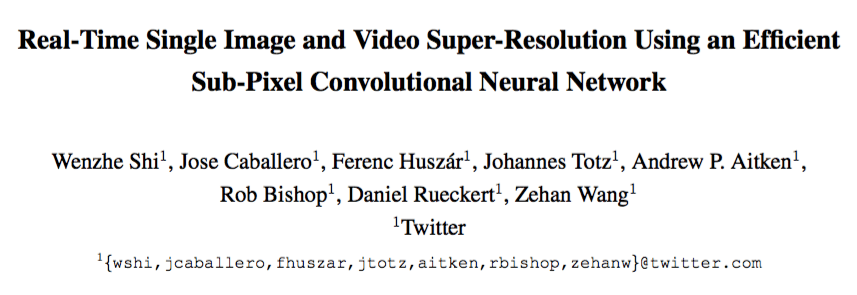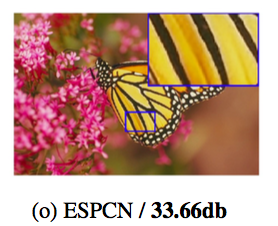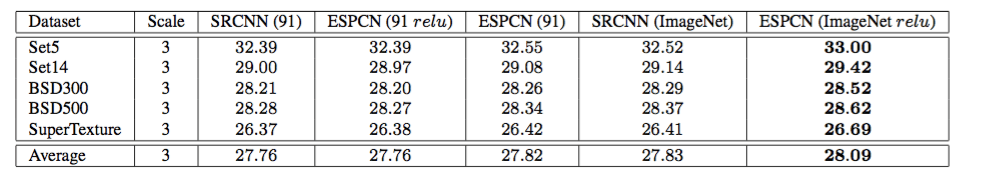# Real-Time Single Image and Video Super-Resolution Using an Efficient Sub-Pixel Convolutional Neural Network

This paper proposed a computationally efficient convolutional layer to upscale the final low-resolution feature map to a high-resolution output.Paper Source:
https://arxiv.org/pdf/1609.05158.pdf
Paper Implementation:
https://github.com/torch/nn/blob/master/PixelShuffle.lua

## Introduction

The goal of Singe Image/Video Super-Resolution is to recover a high-resolution image from a single low-resolution image. The author of this paper proposed a computationally efficient convolutional layer (called sub-pixel convolution layer in this paper) in order to upscale the final low-resolution feature map to a high-resolution output. This way, instead of using handcrafted upscaling filters like the bilinear or bicubic sampler, the sub-pixel convolution layer can learn more complex upscaling operation through training, and the overall computational time is also reduced. All you need to do is define a reconstruction loss (e.g. L2 loss) compared to the original input image/frame, and perform an end-to-end training.The image above is an example of Singe Image Super-Resolution. The left image is based on the bicubic upscaling filter, whereas the right image is based on the proposed model. Obviously, compared to the left one(bicubic filtering), the right one is clearer, and shows less background noise.

Previously, the Single Image Super-Resolution is based on high resolution space, which has several drawbacks. On one hand, if you increase the resolution of the low resolution image, when you are dealing with a convolution operation such as in this case, the computation time will increase. On the other hand, the conversion from low resolution space to high resolution space is based on conventional interpolation methods, which might not bring additional information to solve the ill-posed reconstruction problem.

## Proposed Model

Based on on these drawbacks, the author assumes that if there is any possibility, then we can do a super resolution step based on low-resolution space. Based on that assumption, he proposes a sub-pixel convolution layer. For a network with L layers, they learn n_{L-1} feature maps as they normally do. But for the last one, they do a kind of “pixel shuffle” trick to produce a high resolution output. Then, a more complex low resolution to high resolution mapping will be done. It is a very easy and straightforward idea.The figure shown above is the overall model for low resolution image to high resolution image mapping. As illustrated above, they apply the l-th layer sub-pixel convolution that upscales the I_{LR} feature maps to I_{SR}.The above formula is the mathematical description of the network, where W_l, b_l, l ∈ (1, L – 1) are learnable weights and biases, respectively. W_l is a 2D convolution tensor of size n_{l-1} x n_l x k_l x k_l, where n_{l-1} is the number of feature maps in layer l-1, n_l is the number of filters in layer l, k_l is the filter size, and b_l is the bias vector. The non-linearity function φ is applied element wise.

In the last layer, an upscaling operation occurs. A way to upscale low-resolution image is convolve with stride of 1/r in low-resolution space, with a filter of size k_s and weight spacing 1/r. The convolution operation would activate different parts of that filter, in this case the weights of the filter that fall between pixels are simply not be calculated. To model this operation, the author in this paper propose an mathematical formula:where PS means periodic pixel shuffling, which can rearranges the input tensor of a C * r^2 x H x W to a tensor of shape C x rH x rW. The effects of this operation has been shown in the figure above.

In numpy, we can write it as:Note that the convolution operator W_l has the shape of n_{l-1} x C*r^2 x k_l x k_l and there is no non-linearity at the last layer.
The training objective is based on mean square error (MSE). Given a high resolution training set I_n^{HR}, n=1 … N, they generate the corresponding low resolution images I_n^{LR}, n=1 … N, and after the super-resolution step calculate the pixel wise MSE loss:## Image Super Resolution results

For training, the author selected images from ImageNet . For the data preprocessing, they converted the images from RGB colour space to YCbCr colour space, because human are more sensitive to luminance changes. During the training, 17r x 17r pixel sub-images (e.g. you can choose r = 2, then it becomes 34 x 34) are extracted from original image I^{HR}. The performance metric is based on PSNR.The above figure is the Single Image Super-Resolution results. The proposed model achieved the best PSNR, and the visual comparison of super-resolved images shows that the proposed model creates a much sharper image with higher contrast, and provides noticeable improvements over the other methods.The table above shows the mean PSNB (db) for different dataset. Best results for each category is marked in bold. The proposed model performs better performance than others within these dataset.

## Run time analysis

One of the big advantages of the proposed model is the speed. The author finally evaluate their model on Set14 dataset:As shown above, here is the trade-off between accuracy and speed for different models when performing super-resolution upscaling. Compared to other methods, the above figures shows significant speed-up (> 10x) and better performance (+0.15 dB), which makes it possible to run the proposed model for super-resolution HR videos in real time based on a single GPU.

## Some reviewer’s thoughts

I think the power of single image super resolution is non-trivial to discuss. For example, It can be deployed in the field of video playback, facial recognition, and medical imaging. For HD video playback, you will enjoy it better, and for facial recognition and medical imaging, it can help researcher analyse data. The contribution of this paper is for a simple trick, namely pixel shuffling, to learn a singe image super resolution model. In this case, compared to the previous fixed-size up-sampling kernel, not only could it learn a more complex upsampling function, but also make it extremely fast to run real-time. In the paper, they even showed that based on this technique, it can realize real-time performance for HD-Video super resolution. More importantly, due to efficient pixel shuffling, it could also be directly deployed for 3D convolution, which is important for spatial-temporal video modelling.

## References

 O. Russakovsky, J. Deng, H. Su, J. Krause, S. Satheesh, S. Ma, Z. Huang, A. Karpathy, A. Khosla, M. Bernstein, et al. Imagenet large scale visual recognition challenge. International Journal of Computer Vision, pages 1–42, 2014.

Author: Shawn Yan |Editor: Hao Wang Localized by Synced Global Team: Xiang ChenMachine Intelligence | Technology & Industry | Information & Analysis

## 2 comments on “Real-Time Single Image and Video Super-Resolution Using an Efficient Sub-Pixel Convolutional Neural Network”

1.I am really thankful to the owner of this
website who has shared this fantastic piece of writing at at
this time.

2.